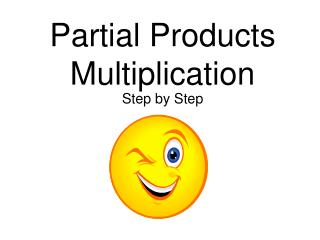DownloadDownload PresentationPartial Products Multiplication

# Partial Products Multiplication

Download Presentation## Partial Products Multiplication

- - - - - - - - - - - - - - - - - - - - - - - - - - - E N D - - - - - - - - - - - - - - - - - - - - - - - - - - -
##### Presentation Transcript

1. Partial ProductsMultiplication Step by Step

2. 742 X 29 Expand each number. 742=700+40+2

3. 742 x 29 Expand each number. 742=700+40+2 29 = 20+9

4. 742 x 29 Multiply 700 x 20. 742=700+40+2 29 = 20+9 700 x 20 = 14000

5. 742 x 29 Multiply 700 x 9. 742=700+40+2 29 = 20+9 700 x 20 = 14000 700 x 9 = 6300

6. 742 x 29 Multiply 40 x 20. 742=700+40+2 29 = 20+9 700 x 20 = 14000 700 x 9 = 6300 40 x 20 = 800

7. 742 x 29 Multiply 40 x 9. 742=700+40+2 29 = 20+9 700 x 20 = 14000 700 x 9 = 6300 40 x 20 = 800 40 x 9 = 360

8. 742 x 29 Multiply 2 x 20. 742=700+40+2 29 = 20+9 700 x 20 = 14000 700 x 9 = 6300 40 x 20 = 800 40 x 9 = 360 2 x 20 = 40

9. 742 x 29 Multiply 2 x 9. 742=700+40+2 29 = 20+9 700 x 20 = 14000 700 x 9 = 6300 40 x 20 = 800 40 x 9 = 360 2 x 20 = 40 2 x 9 = 18

10. 742 x 29 Now, add all you products. 742=700+40+2 29 = 20+9 700 x 20 = 14000 700 x 9 = 6300 40 x 20 = 800 40 x 9 = 360 2 x 20 = 40 2 x 9 = +18 21,518

11. 742 x 29 = 21,518

12. What if there are decimals in the problem???

13. What if there are decimals in the problem??? If your problem has decimals in it, just count the number of digits behind the decimal points in your problem and put that many digits behind the decimal in your answer.

14. What if there are decimals in the problem??? If your problem has decimals in it, just count the number of digits behind the decimal points in your problem and put that many digits behind the decimal in your answer. 35.4 * 3.42 = 121068

15. What if there are decimals in the problem??? If your problem has decimals in it, just count the number of digits behind the decimal points in your problem and put that many digits behind the decimal in your answer. 35.4 * 3.42 = 121068 Where do I put my decimal?

16. What if there are decimals in the problem??? If your problem has decimals in it, just count the number of digits behind the decimal points in your problem and put that many digits behind the decimal in your answer. 35.4 * 3.42 = 121068 Where do I put my decimal? I have 3 digits behind decimals in my problem.. 35.4 * 3.42 = 121068

17. What if there are decimals in the problem??? If your problem has decimals in it, just count the number of digits behind the decimal points in your problem and put that many digits behind the decimal in your answer. 35.4 * 3.42 = 121068 Where do I put my decimal? I have 3 digits behind decimals in my problem.. 35.4 * 3.42 = 121068 So I will have 3 digits behind the decimal in my answer!!! 35.4 * 3.42 = 121.068

18. 35.4 * 3.42 = 121.068 Cool man!! I can’t believe it’s so easy!!!!!!!!!!!